### ANOVA Decomposition

The model that is produced by the multivariate adaptive regression splines algorithm can be formed as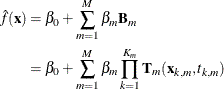Here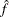is the nonparametric estimate of the response variable in linear models and of the linked response variable in generalized linear models. M is the number of nonconstant bases. For each formed basis,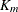is the order of interaction,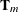is the variable transformation function that depends on the variable type,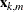is variable for the kth component of the basis, and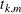is the corresponding knot value or subset categories for the variable.

The function estimate can be recast into the form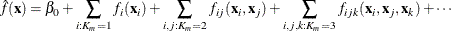where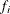represents the sum of bases that involve a single variable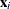,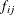represents the sum of bases that involve two-way interactions between transformations of two variables, and so on. The univariate functionis a linear regression spline for variable, which represent the univariate contribution ofto the model. Let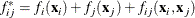Then this bivariate function is a tensor product regression spline that represents the joint contribution by bothand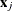. Multivariate functions can be formed similarly if higher-order interaction terms are present in the model. Because of its similarity to the analysis of variance for contingency tables, this is referred as the ANOVA decomposition of the multivariate adaptive regression splines model.